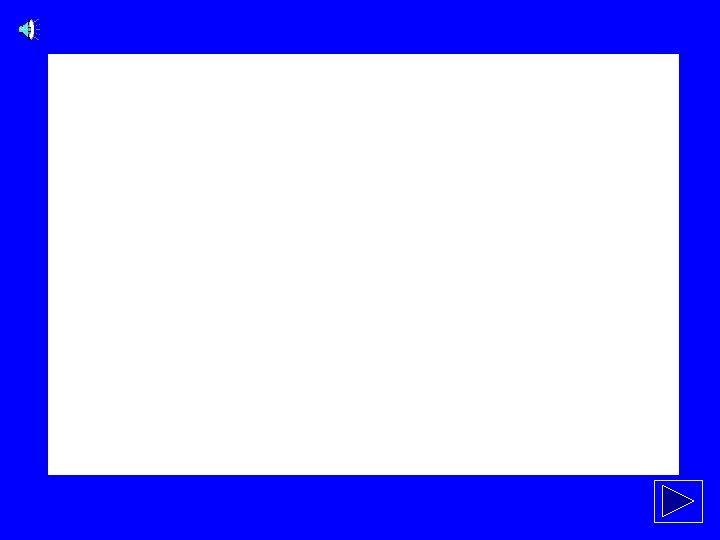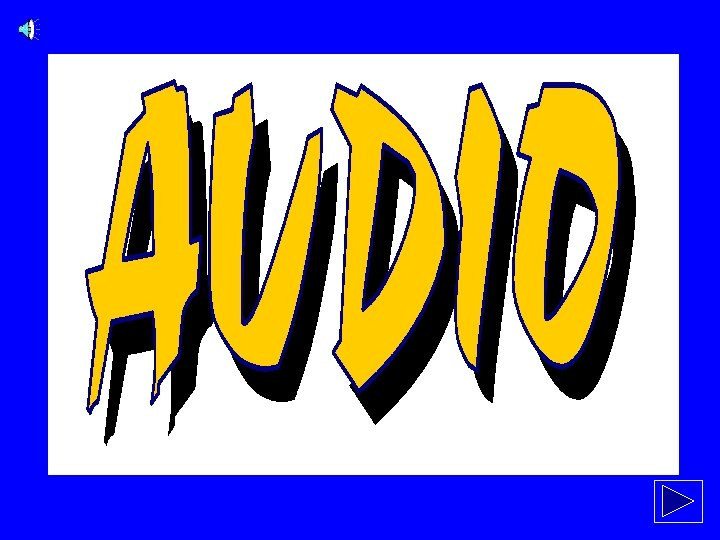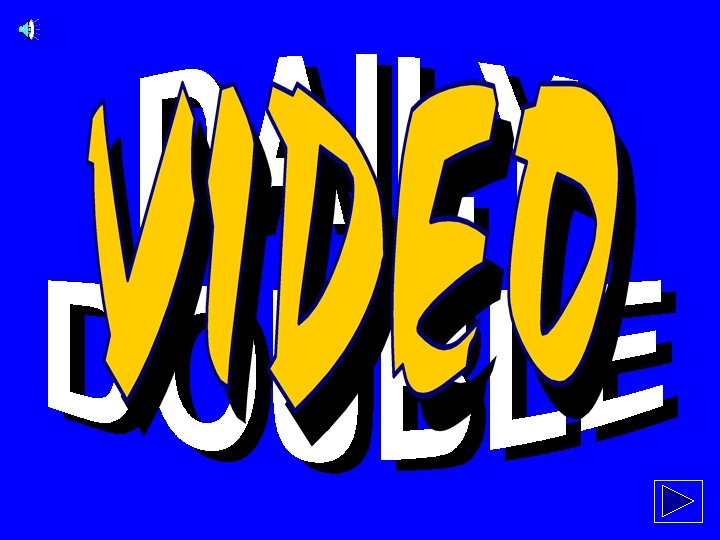# 100 100 200 200 300 300 400 400

• Slides: 67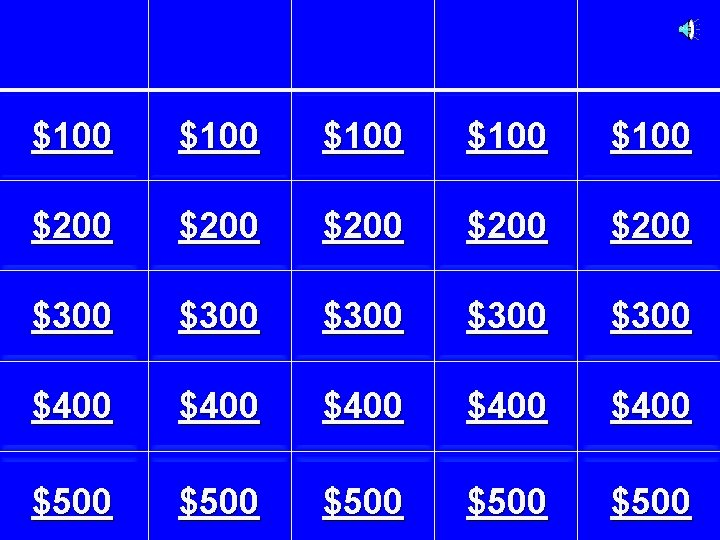\$100 \$100 \$200 \$200 \$300 \$300 \$400 \$400 \$500 \$500Parallel Lines & Perpendicul ar LinesTransverse It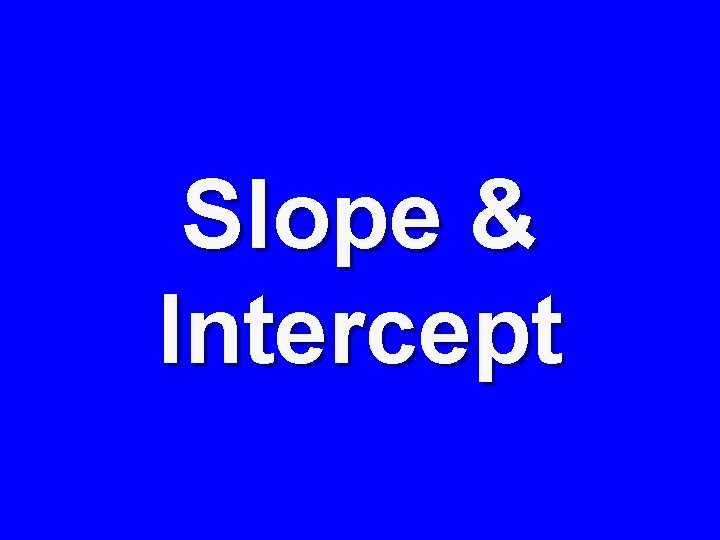Slope & Intercept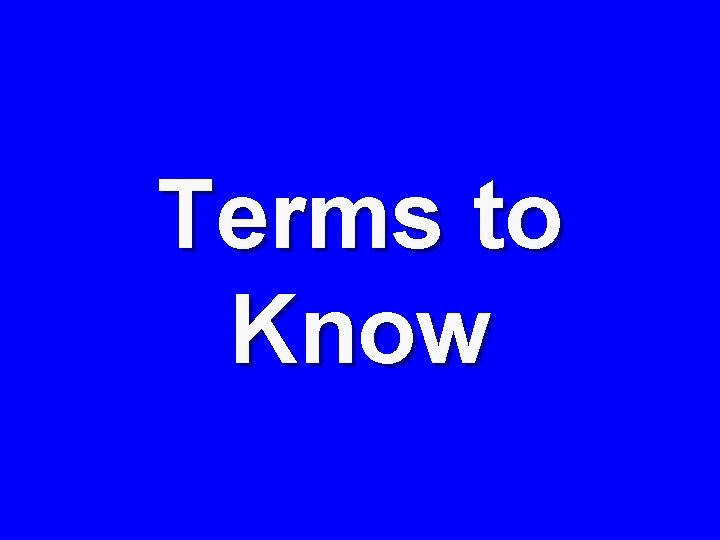Terms to KnowParallel & What’s your Transverse Perpendicu It type? lar Slope & Intercept Terms to Know \$100 \$100 \$200 \$200 \$300 \$300 \$400 \$400 \$500 \$500CATEGORY 1 - \$100 Find the equation of the ling in the graph belowCATEGORY 1 - \$200 The equation of the line that is perpendicular to the y-axis and passes through the point ( 5, -10 )CATEGORY 1 - \$300 The equation of the line that is parallel to 3 y – 6 x = 20 and passes through the point ( -4, 5 )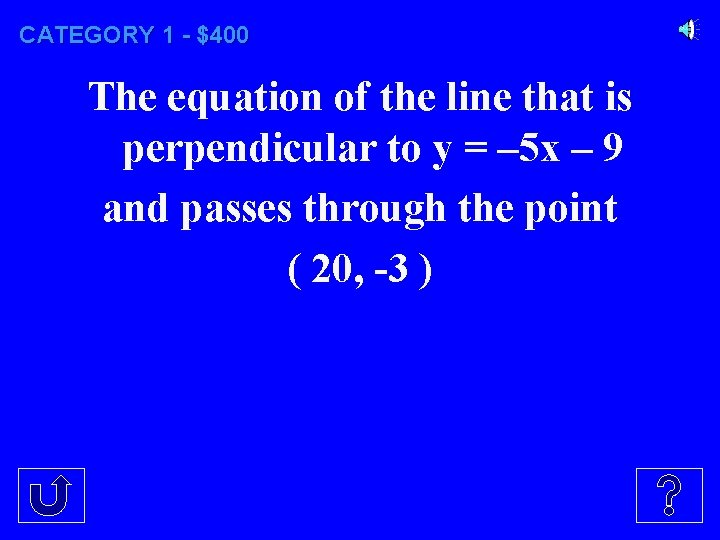CATEGORY 1 - \$400 The equation of the line that is perpendicular to y = – 5 x – 9 and passes through the point ( 20, -3 )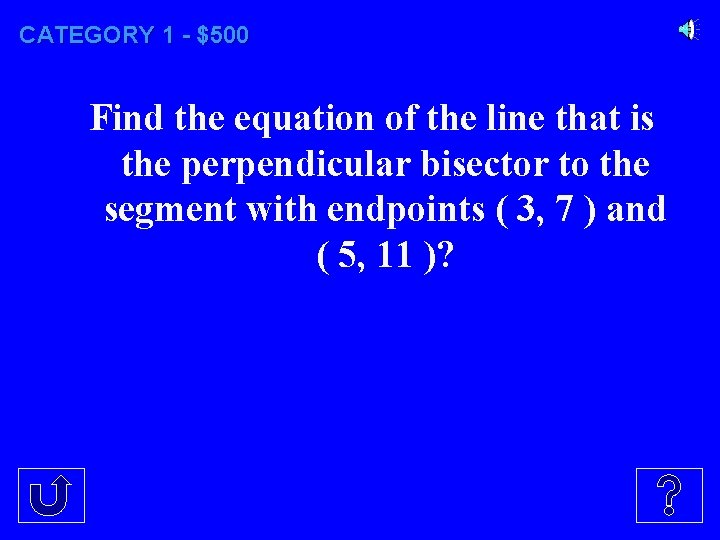CATEGORY 1 - \$500 Find the equation of the line that is the perpendicular bisector to the segment with endpoints ( 3, 7 ) and ( 5, 11 )?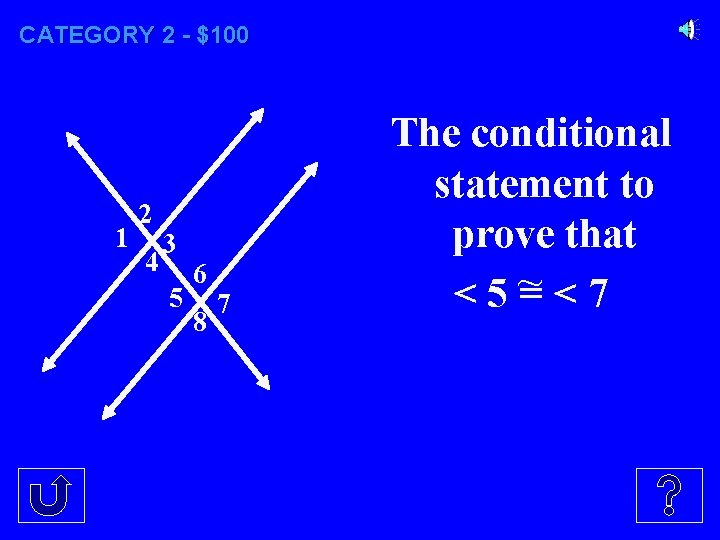CATEGORY 2 - \$100 1 2 4 3 5 6 8 7 The conditional statement to prove that <5~ =<7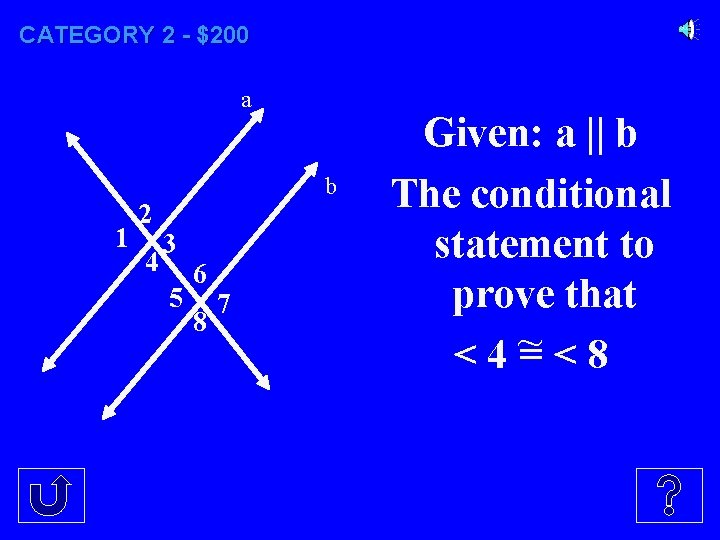CATEGORY 2 - \$200 a 1 2 4 b 3 5 6 8 7 Given: a || b The conditional statement to prove that <4~ =<8CATEGORY 2 - \$300 a 1 2 4 b 3 5 6 8 7 Given: a || b The conditional statement to prove that <2~ =<8CATEGORY 2 - \$400 a 1 2 4 b 3 5 6 8 7 Given: < 3 ~ =<5 The conditional statement to prove that a || b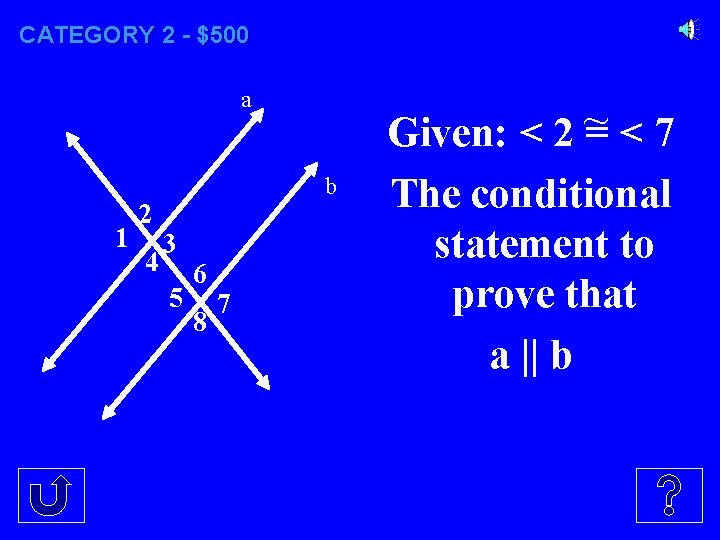CATEGORY 2 - \$500 a 1 2 4 b 3 5 6 8 7 Given: < 2 ~ =<7 The conditional statement to prove that a || bCATEGORY 3 - \$100 a b 1 2 4 3 5 6 8 7 m 5 if a b and m 1 = 95°.CATEGORY 3 - \$200 a b 1 2 4 3 5 6 8 7 m 4 if m 5 = 113°, and a b.CATEGORY 3 - \$300 a b 1 2 4 3 5 6 8 7 m 7 if m 2 = 87°, and a b.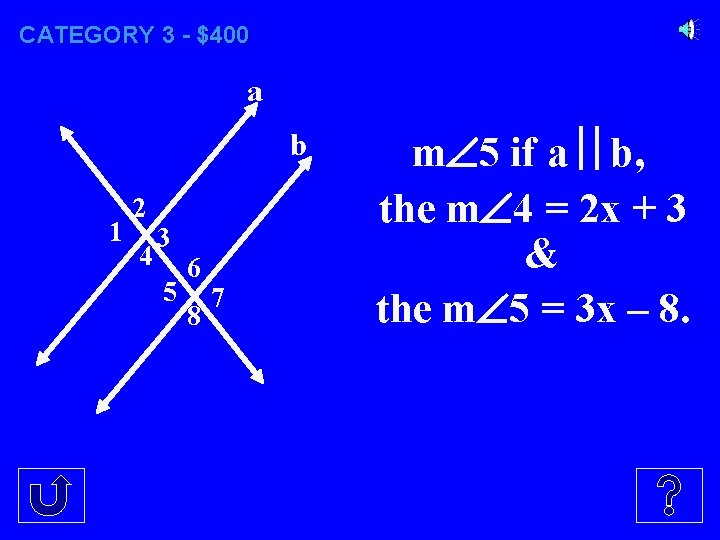CATEGORY 3 - \$400 a b 1 2 4 3 5 6 8 7 m 5 if a b, the m 4 = 2 x + 3 & the m 5 = 3 x – 8.CATEGORY 3 - \$500 a b 1 2 4 3 5 6 8 7 The value of x so that a b, if m 2 = 5 x + 10° & m 6 = 6 x – 4 °.CATEGORY 4 - \$100 The formula for finding the slope of a line between two points.CATEGORY 4 - \$200 The slope of the line containing the points (-3, 2) and (4, 1).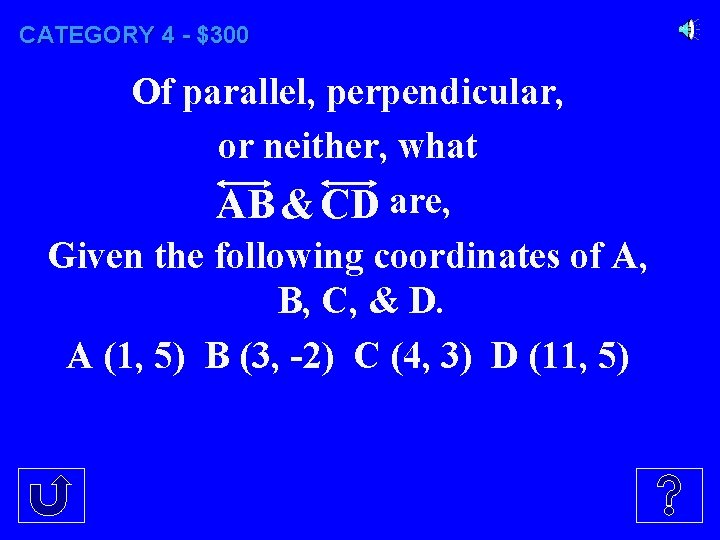CATEGORY 4 - \$300 Of parallel, perpendicular, or neither, what AB & CD are, Given the following coordinates of A, B, C, & D. A (1, 5) B (3, -2) C (4, 3) D (11, 5)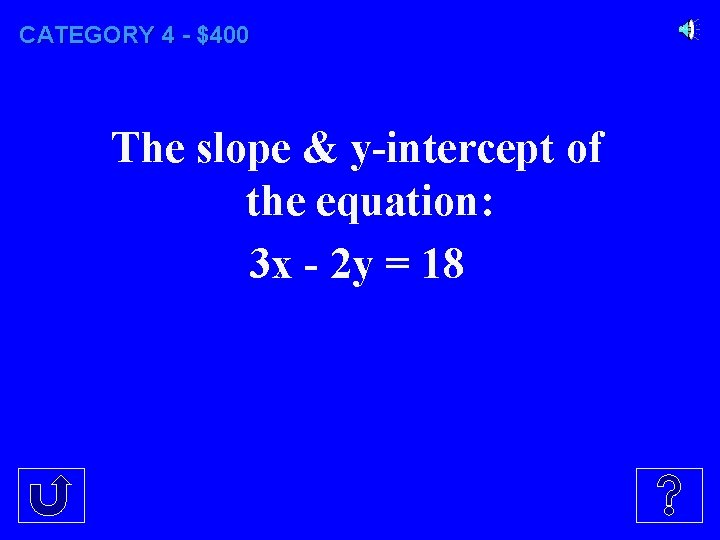CATEGORY 4 - \$400 The slope & y-intercept of the equation: 3 x - 2 y = 18CATEGORY 4 - \$500 The slope of the line that is perpendicular to 4 x – 7 y = 2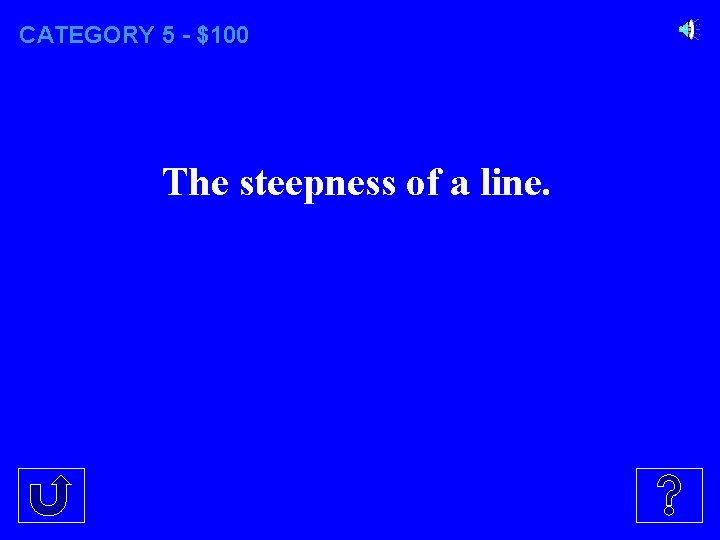CATEGORY 5 - \$100 The steepness of a line.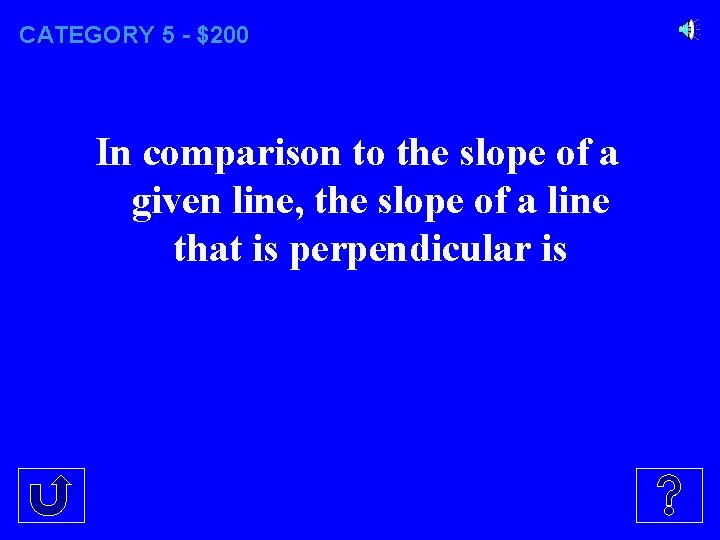CATEGORY 5 - \$200 In comparison to the slope of a given line, the slope of a line that is perpendicular is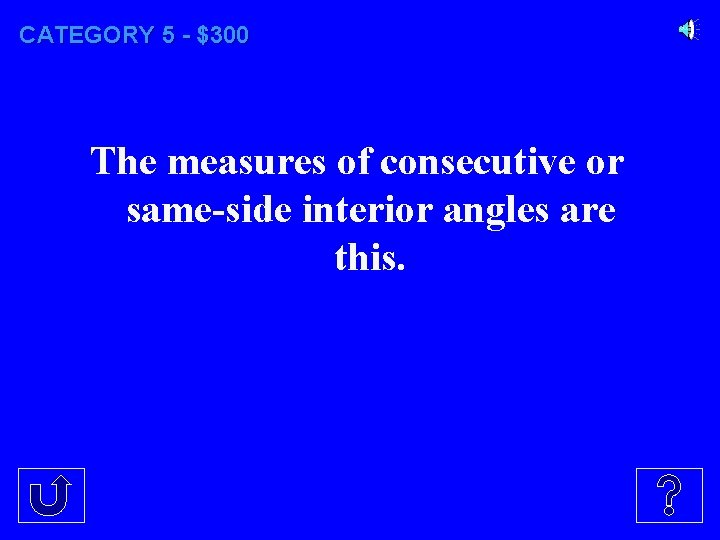CATEGORY 5 - \$300 The measures of consecutive or same-side interior angles are this.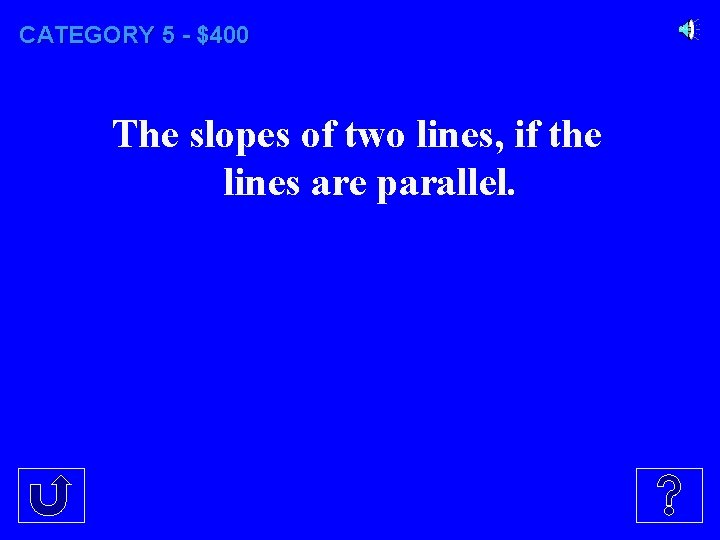CATEGORY 5 - \$400 The slopes of two lines, if the lines are parallel.CATEGORY 5 - \$500 Given a segment with two defined endpoints, the 2 requirements needed in order to find the equation of the line that is the perpendicular bisector of the given segment.CATEGORY 1 - \$100 What is y = -1/4 x – 1?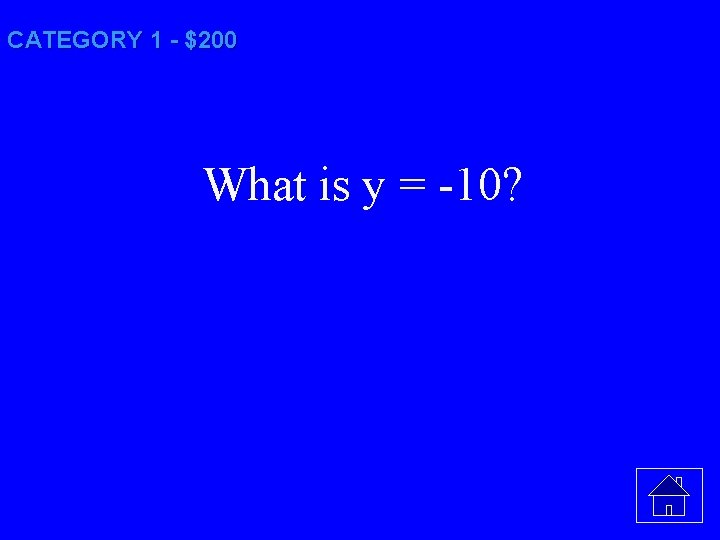CATEGORY 1 - \$200 What is y = -10?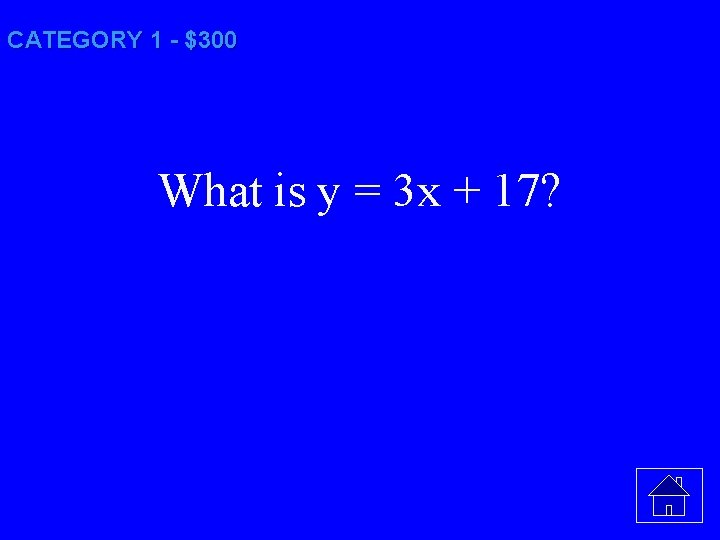CATEGORY 1 - \$300 What is y = 3 x + 17?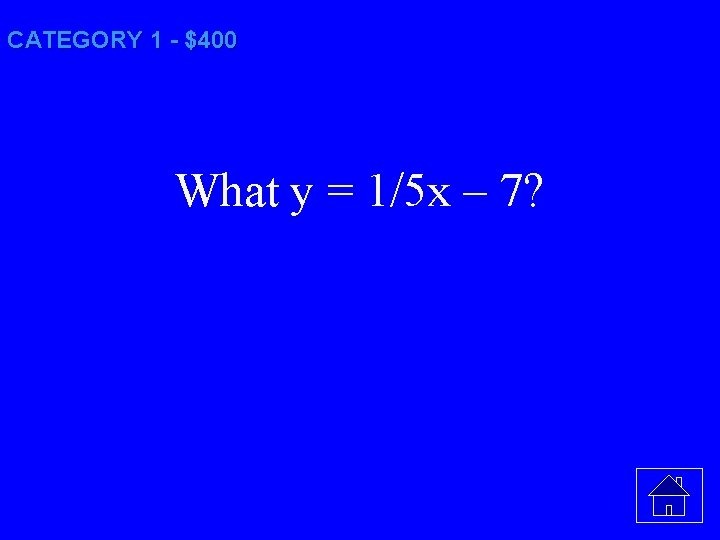CATEGORY 1 - \$400 What y = 1/5 x – 7?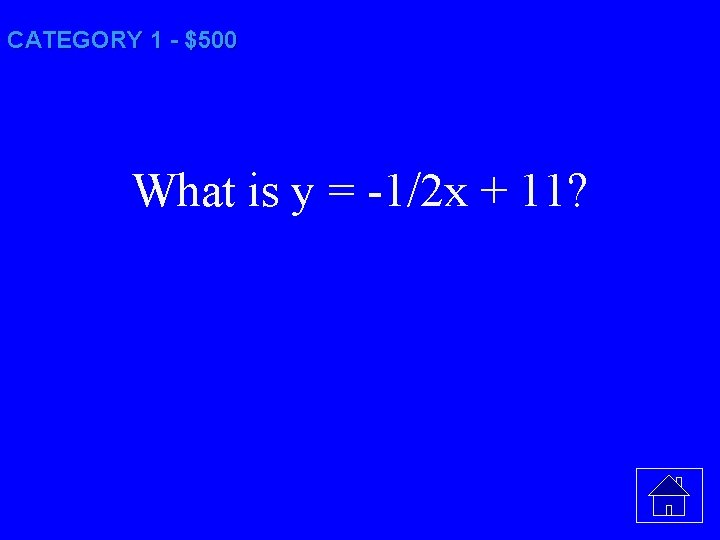CATEGORY 1 - \$500 What is y = -1/2 x + 11?CATEGORY 2 - \$100 What is if two lines intersect then vertical angles are congruent?CATEGORY 2 - \$200 What is if a transversal intersects two parallel lines, then corresponding angles are congruentCATEGORY 2 - \$300 What is if a transversal intersects two parallel lines, then alternate exterior angles are congruentCATEGORY 2 - \$400 What is if two lines and a transversal form alternate interior angles that are congruent then the two lines are parallel?CATEGORY 2 - \$500 What is if two lines and a transversal form same side exterior angles that are supplementary, then the two lines are parallel?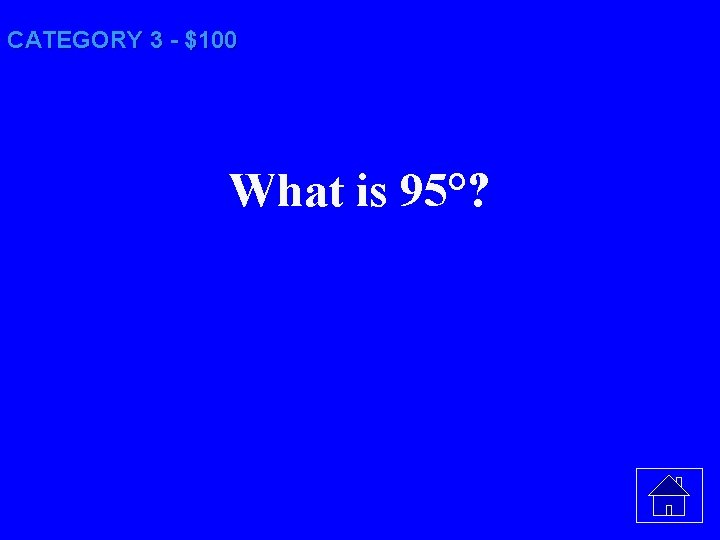CATEGORY 3 - \$100 What is 95°?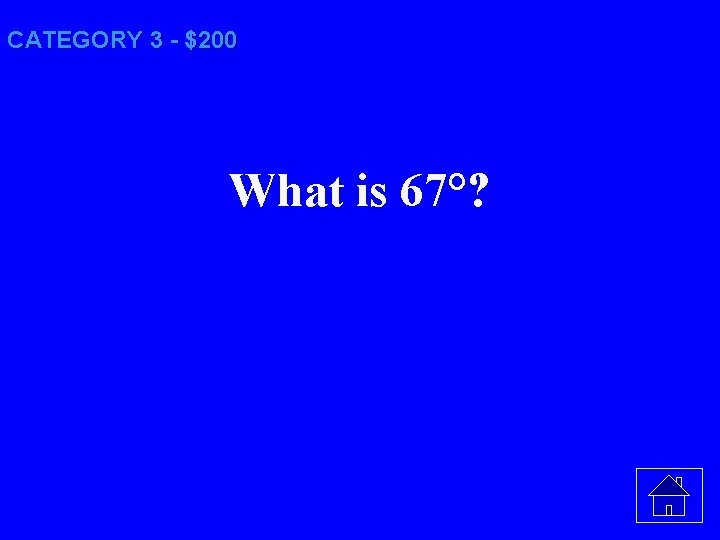CATEGORY 3 - \$200 What is 67°?CATEGORY 3 - \$300 What is 93°?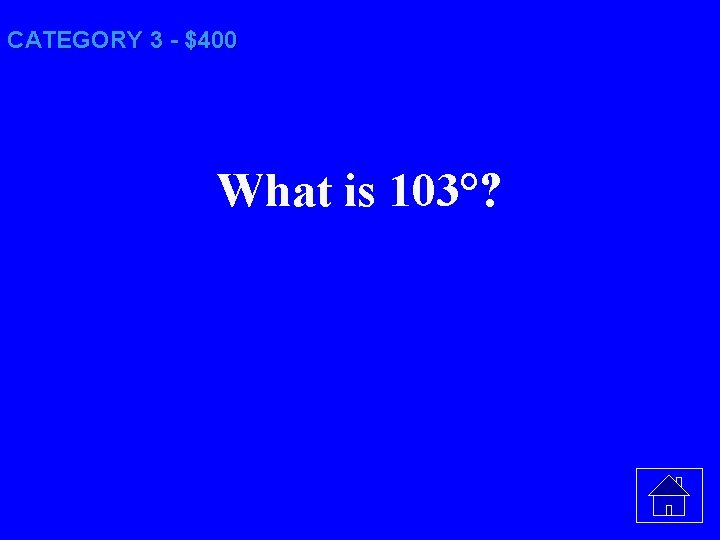CATEGORY 3 - \$400 What is 103°?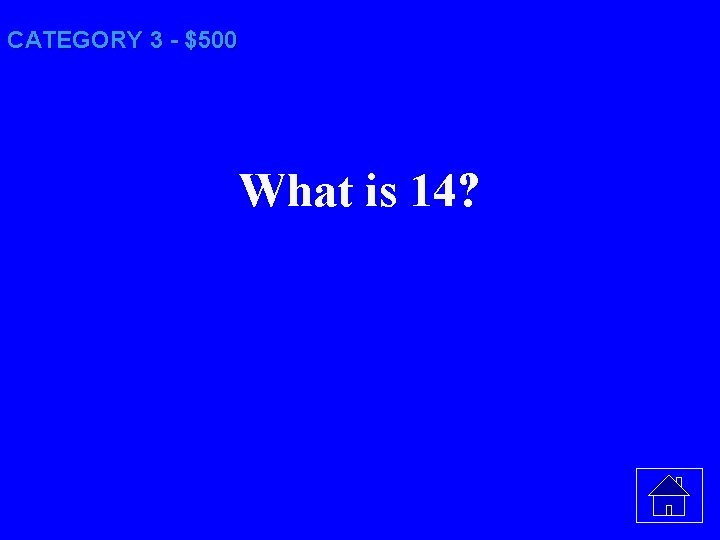CATEGORY 3 - \$500 What is 14?CATEGORY 4 - \$100CATEGORY 4 - \$200 What is – 1/7?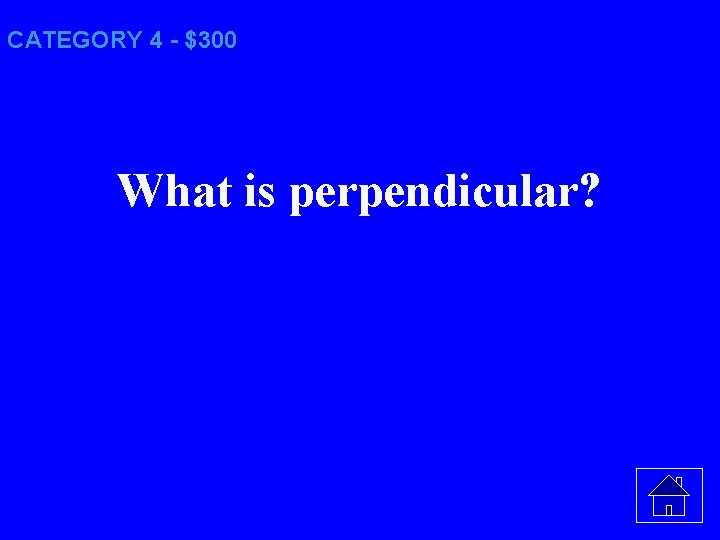CATEGORY 4 - \$300 What is perpendicular?CATEGORY 4 - \$400 What is m = 3/2 and b = -9?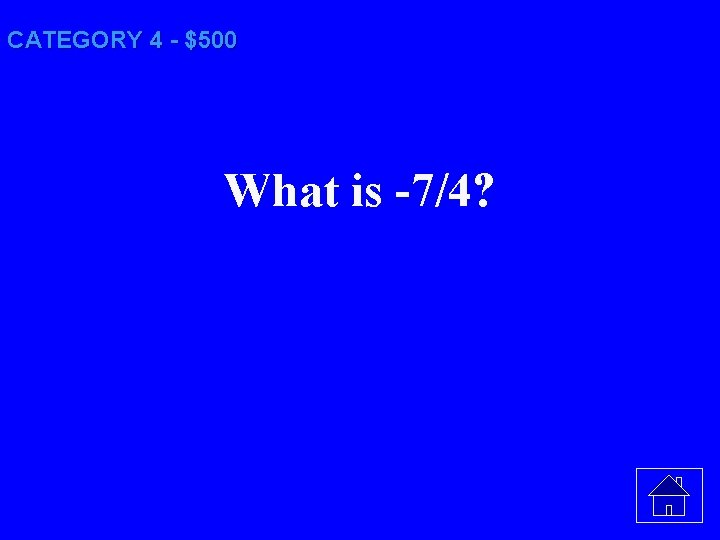CATEGORY 4 - \$500 What is -7/4?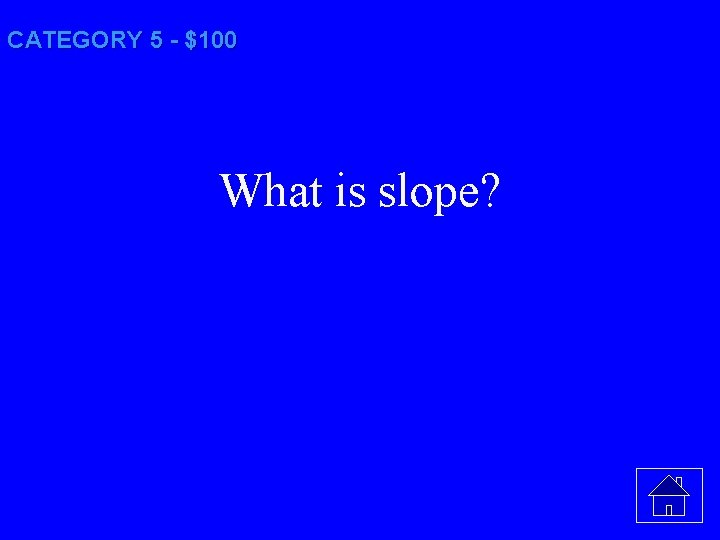CATEGORY 5 - \$100 What is slope?CATEGORY 5 - \$200 What is the negative reciprocal to the slope of the given line?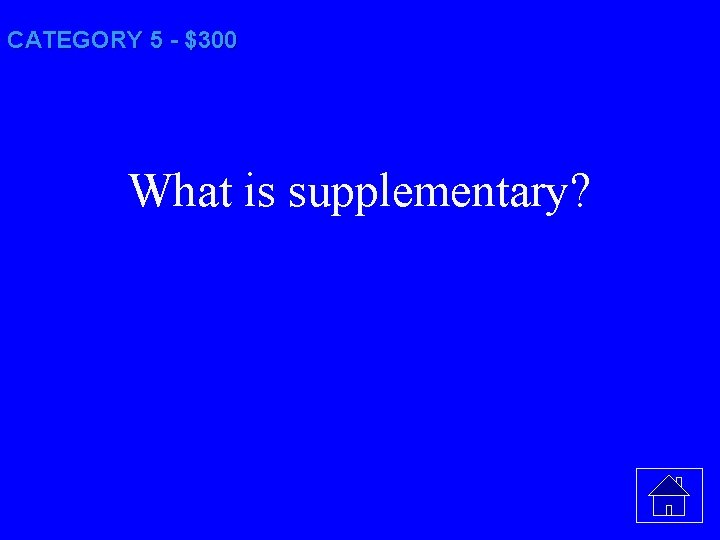CATEGORY 5 - \$300 What is supplementary?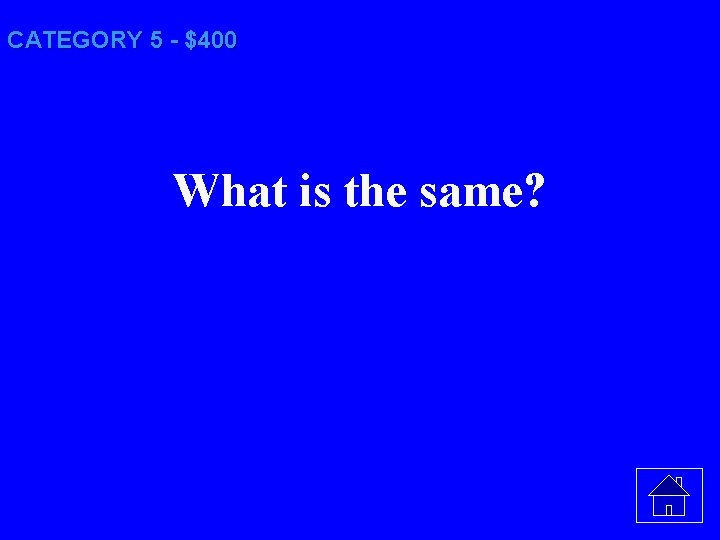CATEGORY 5 - \$400 What is the same?CATEGORY 5 - \$500 What is the midpoint and the negative reciprocal of the slope for the given segmentFinal Category: A Matter of SteepnessFINAL CATEGORY The slope of any line perpendicular to the line y = 3.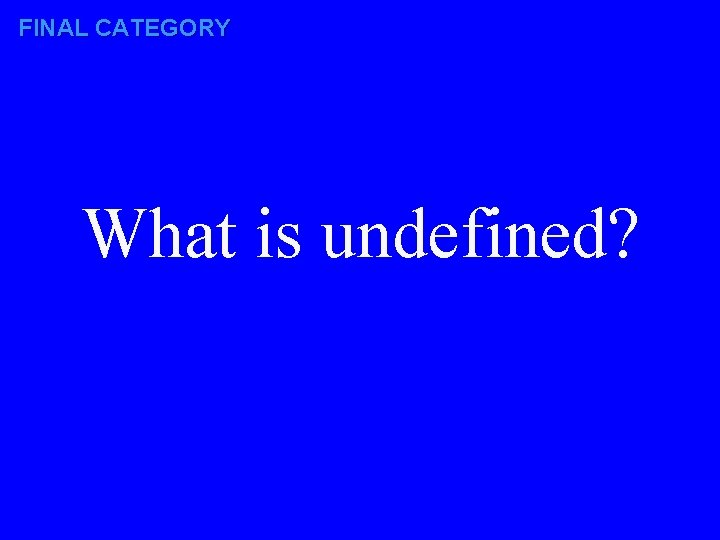FINAL CATEGORY What is undefined?END OF GAME Daily Doubles and usage notes follow. . .